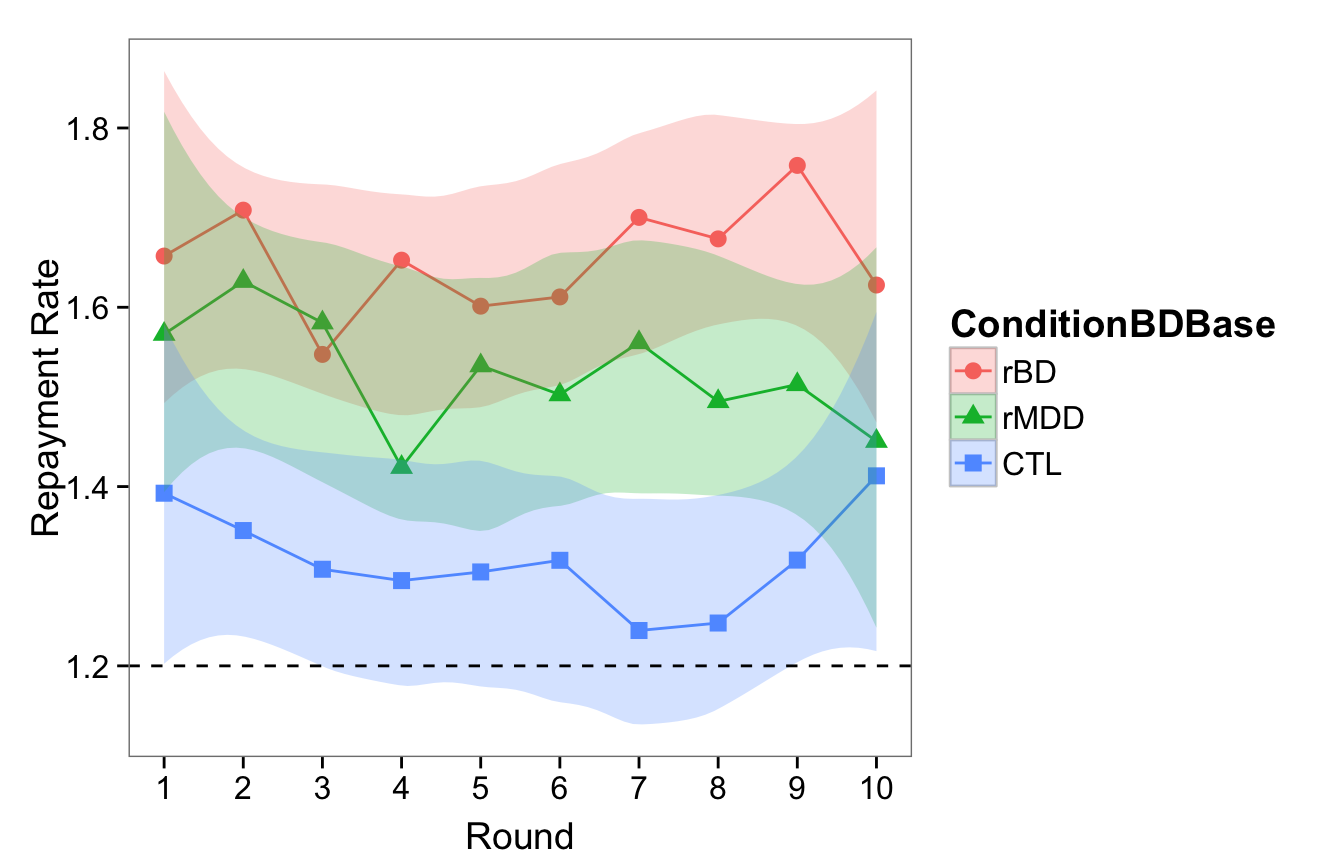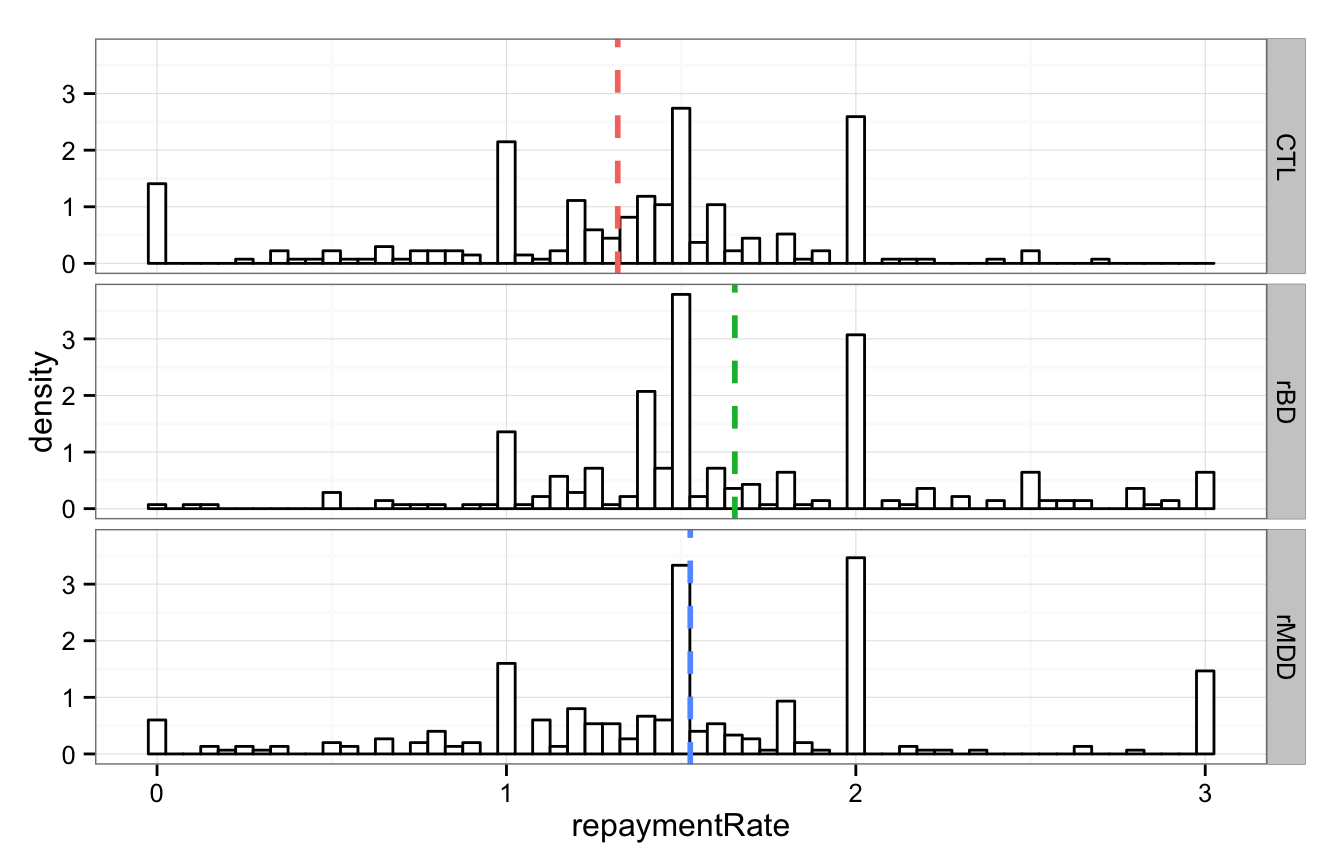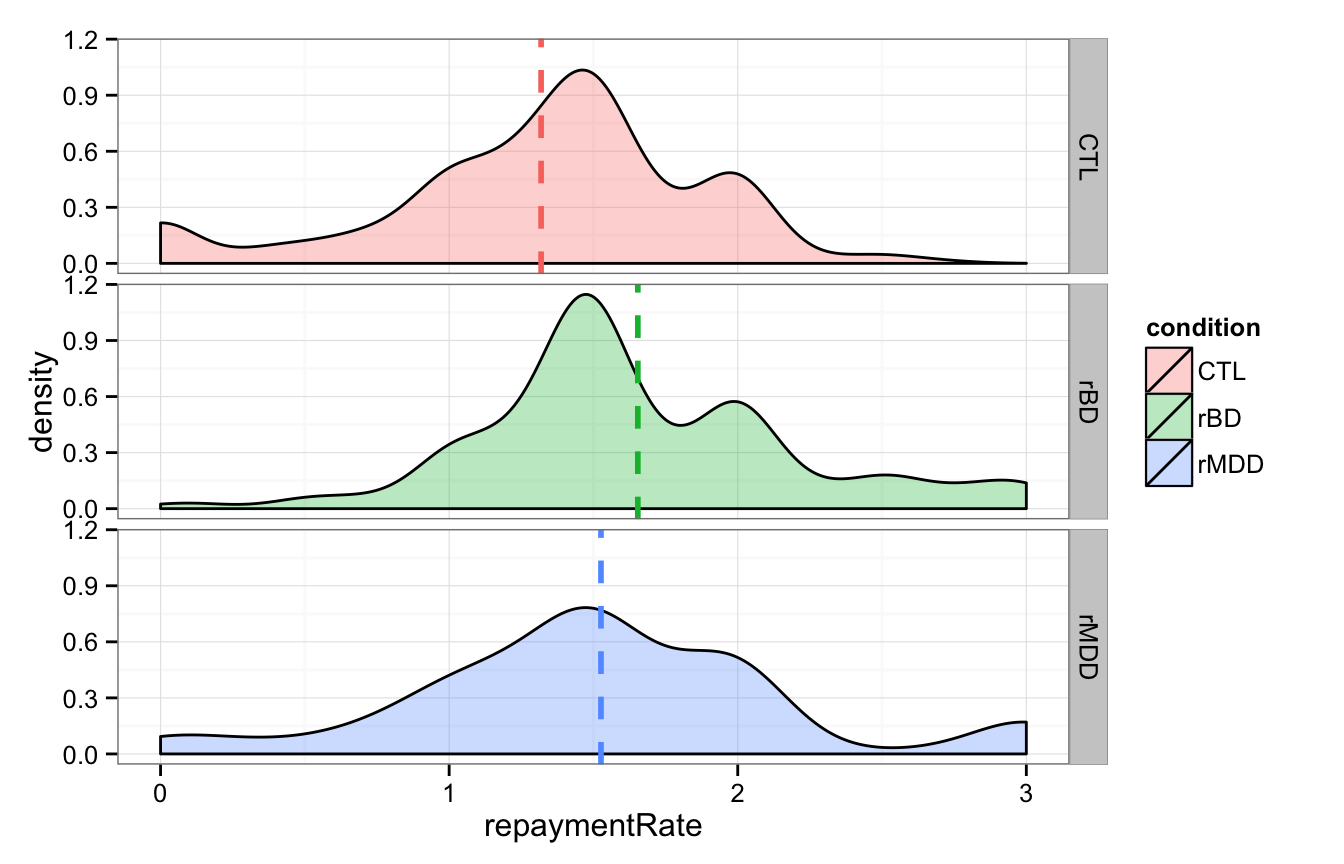# Demographics

The following code outputs Table 1: Demographic and Clinical Characteristics

Descriptives rBD
(n=28)
rMDD
(n=30)
CTL
(n=27)
Statistic
Demographics
Age (Yrs) 29.93 (6.815) 27.87 (6.361) 28.37 (6.541) F=0.7648
Female (%) 64.29% 73.33% 62.96% $$\chi^2$$=0.8372
Caucasian (%) 78.57% 76.67% 74.07% $$\chi^2$$=0.1555
Education (Yrs) 14.35 (1.95) 15.88 (1.67) 15.96 (2.2) F=5.9674
Employed (%) 53.57% 70% 70.37% $$\chi^2$$=2.268
Partnered (%) 42.86% 63.33% 62.96% $$\chi^2$$=3.149
Number Children 1.464 (0.6929) 1.1 (0.4026) 1.333 (0.7338) F=2.5674
Annual Income       $$\chi^2$$=28.95
<$10k 17.86% 13.33% 25.93%$10k-$25k 46.43% 30% 3.704%$26k-$50k 28.57% 23.33% 33.33%$51k-$75k 0% 6.667% 11.11%$76k-$100k 3.571% 20% 0% >$100k 3.571% 6.667% 25.93%

Cognitive
MMSE 28.96 (1.666) 28.43 (1.755) 29.15 (1.379) F=1.5261
WAIS-III 10.82 (3.116) 11.1 (2.881) 10.44 (2.833) F=0.353

Clinical
YMRS 1.321 (1.492) 1.067 (1.202) 0.5556 (0.974) F=2.7026
IDS-C 3.429 (2.332) 4.767 (3.036) 2.296 (2.053) F=6.8289
GAF 70.46 (7.224) 76.97 (7.107) 85.85 (6.407) F=34.0795
Remission Duration (Mos.) 36.95 (36.03) [1 – 135] 31.4 (33.36) [1 – 118]
Age at Onset (Yrs) 18.11 (5.343) 14.5 (3.037) F=10.1647
Illness Duration (Yrs) 12.16 (5.855) 13.27 (5.166) F=0.5837
# Comorbid Disorders 0.1429 (0.3563) 0.4333 (0.6261) F=4.6253
% Comorbid Disorders 14.29% 36.67% $$\chi^2$$=4.441
# Depressive Episodes 13.48 (17.53) 17.23 (24.1) F=0.454
# Manic Episodes 13.62 (21.53)
# Medications 1.464 (1.261) 0.6 (0.7701) F=10.0697
anticonvulsants 39.29% 3.333%
lithium 10.71% 0%
neuroleptics 35.71% 0%
stimulants 0% 3.333%
antidepressants 35.71% 43.33%
benzodiazepines 10.71% 3.333%
sedative-hypnotics and other anxiolytics 14.29% 6.667%

Note: rBD=remitted Bipolar Disorder group; rMDD=remitted Major Depressive Disorder group; CTL=Healthy control group; Employed=Employed full-time or part-time; Partnered=Married or in a relationship; YMRS=Young Mania Rating Scale; IDS-C=Inventory of Depression Symptomatology, Clinician Rating; GAF=Global Assessment of Functioning; Age at Onset=Age of first depressive or manic episode; # Comorbid Disorders=the number of current DSM-IV-TR Axis I comorbidities; % Comorbid Disorders=at least one DSM-IV-TR Axis I comorbidity; # Medications=the number of psychotropic medications currently taken (including anticonvulsants, lithium, neuroleptics, stimulants, antidepressants, benzodiazepines, and sedative-hypnotics and other anxiolytics); Mean values are given with standard deviations in parentheses.

## Trust Game

#### Summary:

• P plays the role of the trustee.
• Investor chooses to invest $X, it gets multipled by 3, P chooses how much of$3X to return.

Investor properties:

• Always start at $5 (mid of endowment) • “Expects” 1.2x investment. If repayment is more than 1.2x, investment on future round will increase; else will decrease. • “Learning Rate” decreases over rounds (i.e. the same relative repayment will affect the program more in earlier rounds than in later rounds) • Learning Rate is double for negative prediction errors (loss aversion) • Minimum investment:$1. (This is so Ps don’t get trapped with no way to repair). This is a very kind hearted investor.
• On round 6, investor will additionally increase his investment by 50% of the difference between his current investment and the max. For example, if current investment is $2, investor will increase it by 50% of (10-2), or$4, to $6. This is also to encourage cooperation. #### In equations, we have: • $$investment(t)$$ $$\equiv$$ Investment on round $$t$$ • $$repayment(t)$$ $$\equiv$$ Repayment (by participant) on round $$t$$ • $$RATIO \equiv$$ Expected Ratio of Repayment/Investment. • Hardcoded to be 1.2 as that’s what the “average” human trustee would return (see meta analysis of Johnson & Mislin, 2011) • $$\alpha_t \equiv$$ Learning Rate on round $$t$$. • $$\alpha_t$$ drawn uniformly from [0.5, 0.75] at $$t=1$$, decreasing to [0.25, 0.5] at $$t=10$$ $investment(1) = 5$ $investment(t+1) = \left\{ \begin{array}{lr} investment(t) + \alpha_t(repayment(t) - RATIO * investment(t)) & : repayment(t) > RATIO * investment(t)\\ investment(t) + 2*\alpha_t(repayment(t) - RATIO * investment(t)) & : repayment(t) < RATIO * investment(t) \end{array} \right.$ Additional “boost” on round 6 (in addition to the earlier equation) $investment(6) \quad += .5*(10 - investment(5))$ Capping investment (minimum and maximum values are 1 and 10 respectively) $investment(t) = \max(\min(investment(t), 10), 1)$ #### In code, we have: ## This section contains the relevant excerpt from the MATLAB code for the experiment. ## The full Matlab file is available on the Github repository. MULTIPLIER = 3; expectedValueRatio = 1.2; % --- deciding investment amount --- % %Variable decreasing learning rate: %for positive PE, uniformly drawn between [0.5 and 0.75] on first round to [0.25 and 0.5] on last round %for negative PE, uniformly drawn between [1.00 and 1.25] on each round to [0.5 to 0.75] on last round learningRateLowerBound = (trialNumber*(.25) + (10-trialNumber)*(.5))/10; randProp = rand(); if (trialNumber==1) investments(trialNumber)=5; % start with initial investment of 5. else if((repayments(trialNumber-1) - expectedValueRatio*investments(trialNumber-1)) >0) learningRate(trialNumber) = learningRateLowerBound + (randProp * .25) else learningRate(trialNumber) = 2*learningRateLowerBound + (randProp * .25) end if (trialNumber==6) % Boost investments(trialNumber) = investments(trialNumber-1) + ... learningRate(trialNumber)*(repayments(trialNumber-1) - expectedValueRatio*investments(trialNumber-1)) +... .5*(10 - investments(trialNumber-1)); else investments(trialNumber) = investments(trialNumber-1) + ... learningRate(trialNumber)*(repayments(trialNumber-1) - expectedValueRatio*investments(trialNumber-1)); end end % Cap it between 0 and 10 investments(trialNumber) = max(min(investments(trialNumber),10),1); % Round to nearest 0.50 investments(trialNumber) = round(investments(trialNumber)*2)/2; investment=investments(trialNumber); ## Results ### Average repayment rate Because how much the trustee chooses to return ($0-$3X) depends on how much gets invested ($X), we defined a repayment rate as a proportion of the amount investment. So repayment rate goes from 0-3. Below are the mean repayment rates as a function of the round played. (The “amount the investor expects” is 1.2, plotted as a dashed line).

As you can see, the average repayment rate is very high! People tended to default to cooperation. And the average rMDD and rBD repayment rates are higher than the control (rMDD > CTL is not significant, rBD > CTL is significant). (More in the modeling section below.)

Below that graph are more fine-grained histograms:### Modeling

The models we fitted were simple linear models predicting repaymentRate (how much the participant repaid / how much the participant was entrusted with).

First, we modeled the interaction of “round” and clinical history, but turns out that “round” has no significant interactions with condition nor any simple effect ($$\chi^2(3)$$=2.2447; p=0.5232). That means that on average, individuals tended to keep the same repayment rate over the consecutive rounds of the game.

Next, we fit a simple mixed effects model predicting the repayment rate with the clinical histroy (“condition”) as a fixed effect, and random intercepts and slopes (on round) by participant. We see that on average, both rBD and rMDD participants had a higher repayment rate than CTL participants. There was no difference between rMDD and CTL.

The following model has a marginal $$R^2$$ of 0.0491 and a conditional $$R^2$$ of 0.6772, after Nakagawa & Schielzeth (2013).

lmer(repaymentRate ~ condition + (1 + round | subjectID), tgData)
Regressor b (SE) 95% CI t p
rBD (> CTL) 0.3033 (0.1247) [0.0588, 0.5478] t(81.9819)=2.4313 p=0.0172
rMDD (> CTL) 0.2407 (0.1227) [2.2756 × 10-4, 0.4812] t(81.9819)=1.9619 p=0.0532

rBD (> rMDD) 0.0626 (0.1215) [-0.1756, 0.3008] t(81.9819)=0.5151 p=0.6078

Top: Predicting repayment rate. Condition is a 3 level categorical variable: the top two lines are the values of the coefficients in the same model with CTL as the base, comparison group. The last line is the same model but with the categorical variable releveled to have rMDD as the base, comparison group, in order to examine the rBD>rMDD contrast.

### Summary statistics across rounds

Next, we calculated four summary statistics:

• the total amount that the investor program invested in the participant,
• the total amount that the participant repaid,
• the total amount that the participant kept across all ten rounds,
• and the total amount that the investor made across all ten rounds.

Note that these are all correlated, and also correlated with the repaymentRate, especially since the investor program is deterministic (up to a pseudo-random learning rate).

As expected, we find that rBD > CTL for total amount invested, and total amount repaid, but not for total amount made in total. None of the other comparisons (rMDD > CTL or rBD > rMDD) turned out significant.

Regressors b (SE) 95% CI t p
Predicting Total Amount Invested by Investor
rBD (> CTL) 12.414 (6.0073) [0.6397, 24.1884] t=2.0665 p=0.0419
rMDD (> CTL) 5.9426 (5.9082) [-5.6375, 17.5227] t=1.0058 p=0.3175
$$R^2$$ 0.0496

Predicting Total Amount Repaid by Participant
rBD (> CTL) 34.7212 (16.2532) [2.8649, 66.5776] t=2.1363 p=0.0356
rMDD (> CTL) 22.1944 (15.9851) [-9.1363, 53.5252] t=1.3884 p=0.1688
$$R^2$$ 0.0539

Predicting Total Amount Kept by Participant
rBD (> CTL) 2.5208 (8.2809) [-13.7097, 18.7514] t=0.3044 p=0.7616
rMDD (> CTL) -4.3667 (8.1443) [-20.3294, 11.5961] t=-0.5362 p=0.5933
$$R^2$$ 0.0091

Predicting Total Amount Kept by Investor
rBD (> CTL) 22.3072 (11.2603) [0.2371, 44.3774] t=1.9811 p=0.0509
rMDD (> CTL) 16.2519 (11.0745) [-5.4541, 37.9578] t=1.4675 p=0.1461
$$R^2$$ 0.0486